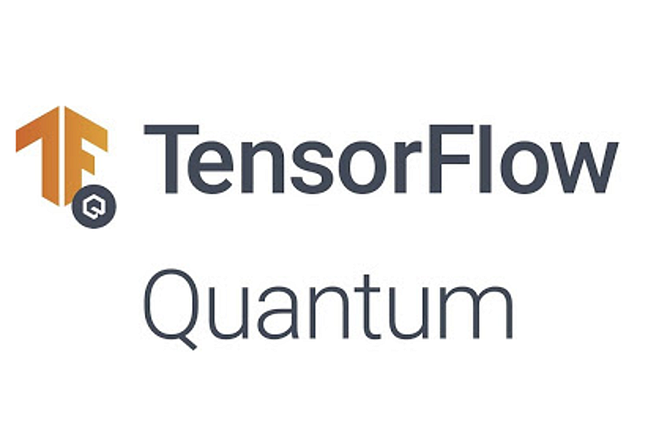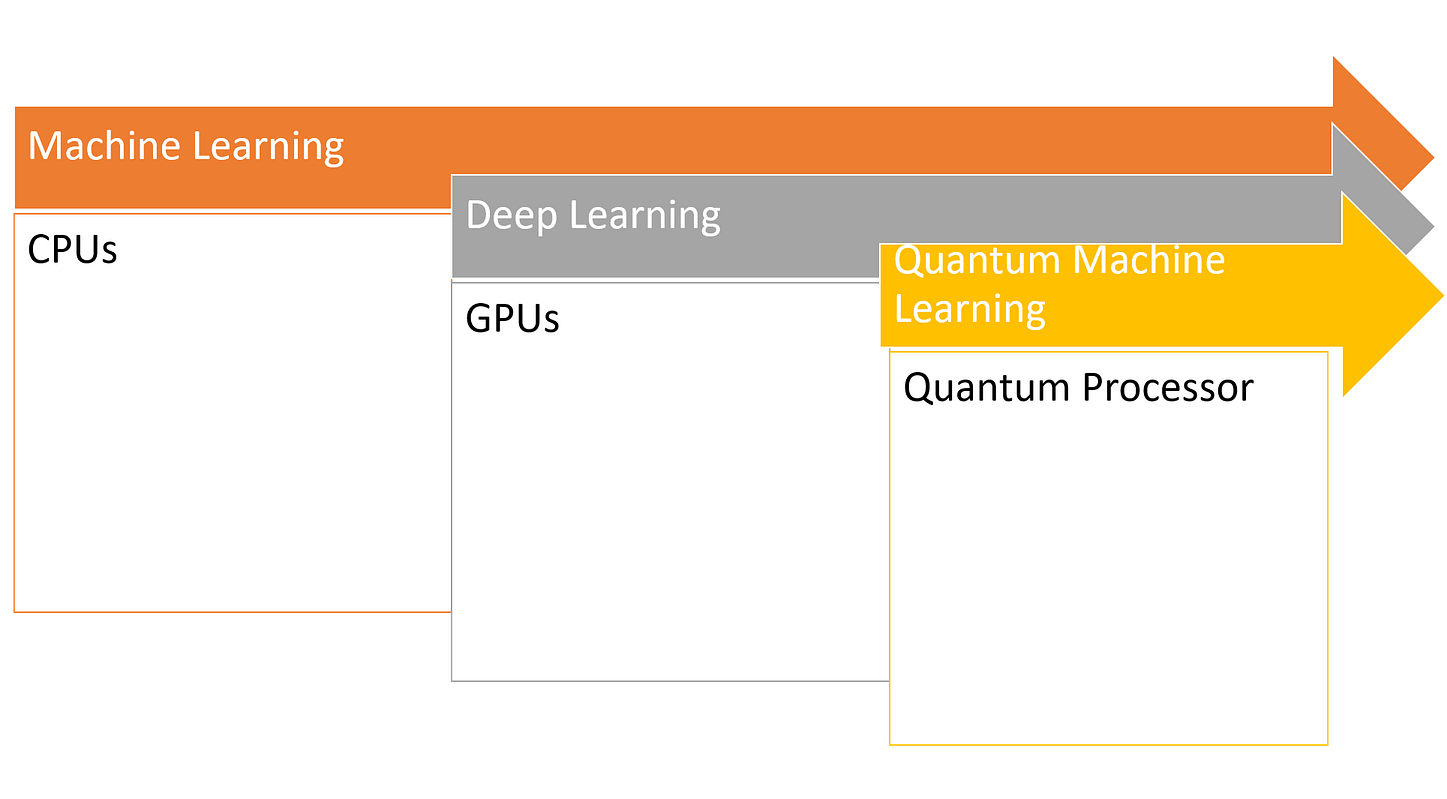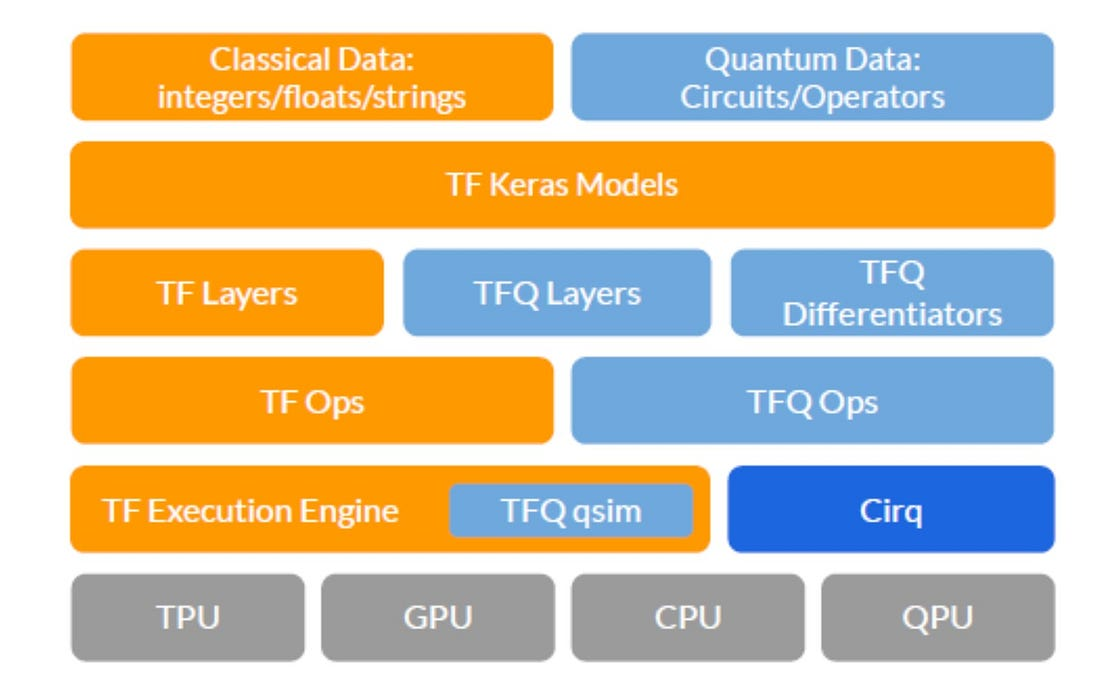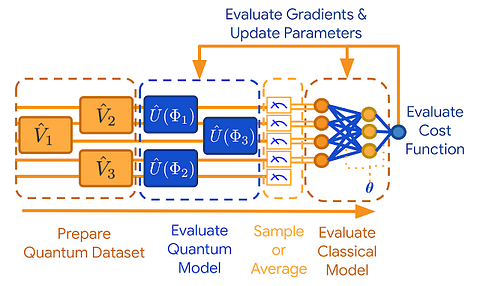Submit a blog to KDnuggets -- Top Blogs Win A Reward

Topics: AI | Data Science | Data Visualization | Deep Learning | Machine Learning | NLP | Python | R | Statistics

KDnuggets Home » News » 2020 » Mar » Tutorials, Overviews » Exploring TensorFlow Quantum, Google’s New Framework for Creating Quantum Machine Learning Models ( 20:n12 )

# Exploring TensorFlow Quantum, Google’s New Framework for Creating Quantum Machine Learning Models

TensorFlow Quantum allow data scientists to build machine learning models that work on quantum architectures.The intersection of quantum computing and artificial intelligence(AI) promises to be one of the most fascinating movement in the entire history of technology. The emergence of quantum computing its likely to force us to reimagine almost all the existing computing paradigms and AI is not an exception. However, the computational power of quantum computers also has the potential to accelerate many areas of AI that remain unpractical today. The first step for AI and quantum computing to work together is to reimagine machine learning models to work on quantum architectures. Recently, Google open sourced TensorFlow Quantum, a framework for building quantum machine learning models.

The core idea of TensorFlow Quantum is to interleave quantum algorithms and machine learning programs all within the TensorFlow programming model. Google refers to this approach as quantum machine learning and is able to implement it by leveraging some of its recent quantum computing frameworks such as Google Cirq.

### Quantum Machine Learning

The first question that we need to answer when comes to quantum computing and AI is how the latter can benefit from the emergence of quantum architectures. Quantum Machine Learning(QML) is a broad term to refer to machine learning models that can leverage quantum properties. The first QML applications focused on refactoring traditional machine learning models so they were able to perform fast linear algebra on a state space that grows exponentially with the number of qubits. However, the evolution of quantum hardware have expanded the horizons of QML evolving onto heuristic methods which can be studied empirically due to the increased computational capability of quantum hardware. This process is analogous to how the creation of GPUs made machine learning evolved towards the deep learning paradigm.In the context of TensorFlow Quantum, QML can be defined as two main components:

1. Quantum Datasets
2. Hybrid Quantum Models

### Quantum Datasets

Quantum data is any data source that occurs in a natural or artificial quantum system. This can be the classical data resulting from quantum mechanical experiments, or data which is directly generated by a quantum device and then fed into an algorithm as input. There is there is some evidence that hybrid quantum-classical machine learning applications on “quantum data” could provide a quantum advantage over classical-only machine learning for reasons described below. Quantum data exhibits superposition and entanglement, leading to joint probability distributions that could require an exponential amount of classical computational resources to represent or store.

### Hybrid Quantum Models

Just like machine learning can generalize models from training datasets, QML would be able to generalize quantum models from quantum datasets. However, because quantum processors are still fairly small and noisy, quantum models cannot generalize quantum data using quantum processors alone. Hybrid quantum models proposes a scheme in which quantum computers will be most useful as hardware accelerators, working in symbiosis with traditional computers. This model is perfect for TensorFlow since it already supports heterogeneous computing across CPUs, GPUs, and TPUs.

### Cirq

The first step to build hybrid quantum models is to be able to leverage quantum operations. In order to do that, TensorFlow Quantum relies on Cirq, an open-source framework for invoking quantum circuits on near term devices. Cirq contains the basic structures, such as qubits, gates, circuits, and measurement operators, that are required for specifying quantum computations. The idea behind Cirq is to provide a simple programming model that abstracts the fundamental building blocks of quantum applications. The current version includes the following key building blocks:

• Circuits: In Cirq, a Cirquit represents the most basic form of a quantum circuit. A Cirq Circuit is represented as a collection of Moments which include operations that can be executed on Qubits during some abstract slide of time.
• Schedules and Devices: A Schedule is another form of quantum circuit that includes more detailed information about the timing and duration of the gates. Conceptually, a Schedule is made up of a set of ScheduledOperations as well as a description of the Device on which the schedule is intended to be run.
• Gates: In Cirq, Gates abstract operations on collections of qubits.
• Simulators: Cirq includes a Python simulator that can be used to run Circuits and Schedules. The Simulator architecture can scale across multiple threads and CPUs which allows it to run fairly sophisticated Circuits.

### TensorFlow Quantum

TensorFlow Quantum(TFQ) is a framework for building QML applications. TFQ allows machine learning researchers to construct quantum datasets, quantum models, and classical control parameters as tensors in a single computational graph.
From an architecture standpoint, TFQ provides a model that abstracts the interactions with TensorFlow, Cirq, and computational hardware. At the top of the stack is the data to be processed. Classical data is natively processed by TensorFlow; TFQ adds the ability to process quantum data, consisting of both quantum circuits and quantum operators. The next level down the stack is the Keras API in TensorFlow. Since a core principle of TFQ is native integration with core TensorFlow, in particular with Keras models and optimizers, this level spans the full width of the stack. Underneath the Keras model abstractions are our quantum layers and differentiators, which enable hybrid quantum-classical automatic differentiation when connected with classical TensorFlow layers. Underneath the layers and differentiators, TFQ relies on TensorFlow ops, which instantiate the dataflow graph.In terms of execution standpoint, TFQ follows the following steps to train and build QML models.

1. Prepare a quantum dataset: Quantum data is loaded as tensors, specified as a quantum circuit written in Cirq. The tensor is executed by TensorFlow on the quantum computer to generate a quantum dataset.
2. Evaluate a quantum neural network model: In this step, the researcher can prototype a quantum neural network using Cirq that they will later embed inside of a TensorFlow compute graph.
3. Sample or Average: This step leverages methods for averaging over several runs involving steps (1) and (2).
4. Evaluate a classical neural networks model : This step uses classical deep neural networks to distill such correlations between the measures extracted in the previous steps.
5. Evaluate Cost Function: Similar to traditional machine learning models, TFQ uses this step to evaluate a cost function. This could be based on how accurately the model performs the classification task if the quantum data was labeled, or other criteria if the task is unsupervised.
6. Evaluate Gradients & Update Parameters — After evaluating the cost function, the free parameters in the pipeline should be updated in a direction expected to decrease the cost.The combination of TensorFlow and Cirq enable TFQ with a rick set of capabilities including a simpler and familiar programming model as well as the ability to simultaneously train and execute many quantum circuits.

The efforts related to bridging quantum computing and machine learning are still in very nascent stages. Certainly, TFQ represents one of the most important milestones in this area and one that leverages some of the best IP in both quantum and machine learning. More details about TFQ can be found in the project’s website.

Original. Reposted with permission.

Related:

## Top Stories Past 30 Days

Most Popular
Most SharedGet KDnuggets, a leading newsletter on AI, Data Science, and Machine Learning

By subscribing you accept KDnuggets Privacy Policy